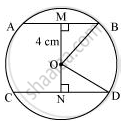Share

# The lengths of two parallel chords of a circle are 6 cm and 8 cm. If the smaller chord is at distance 4 cm from the centre, what is the distance of the other chord from the centre? - CBSE Class 9 - Mathematics

#### Question

The lengths of two parallel chords of a circle are 6 cm and 8 cm. If the smaller chord is at distance 4 cm from the centre, what is the distance of the other chord from the centre?

#### SolutionLet AB and CD be two parallel chords in a circle centered at O. Join OB and OD.

Distance of smaller chord AB from the centre of the circle = 4 cm

OM = 4 cm

MB = AB/2 = 6/2 = 3cm

In ΔOMB,

OM2 + MB2 = OB2

(4)2 + (3)2 = OB2

16 + 9 = OB2

OB2 = 25

OB = sqrt25

OB = 5cm

In ΔOND,

OD = OB = 5cm                   (Radii of the same circle)

ND = CD/2 = 8/2 = 4cm

ON2 + ND2 = OD2

ON2 + (4)2 = (5)2

ON2 = 25 - 16 = 9

ON = 3

Therefore, the distance of the bigger chord from the centre is 3 cm.

Is there an error in this question or solution?

#### APPEARS IN

NCERT Solution for Mathematics Class 9 (2018 to Current)
Chapter 10: Circles
Ex. 10.60 | Q: 3 | Page no. 187

#### Video TutorialsVIEW ALL 

Solution The lengths of two parallel chords of a circle are 6 cm and 8 cm. If the smaller chord is at distance 4 cm from the centre, what is the distance of the other chord from the centre? Concept: Cyclic Quadrilaterals.
S